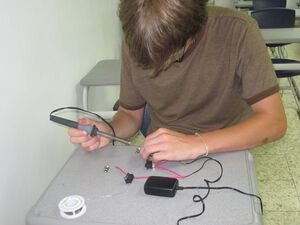After circuit has been verified complete start soldering wires together.

The purpose of this electricity module is to create a portable and inexpensive tool to teach students about circuits in parallel and in series, the two simplest ways to connect a circuit. This knowledge can then be applied to a variety of renewable energy projects. Two or more boxes are constructed to demonstrate how series and parallel circuits interact. Students apply Ohm's law to calculate the flowing current at any section in the circuit with the voltage and resistance.

Ohm's Law where:

$I=Current(Amps)$$V=Voltage(Volts)$$R=Resistance(Ohms)$$I=V/R$## Materials

Material Quantity Total Price
Wire/Alambre 20 ft \$67 (RD) / \$1.67 (US)
Scissors/Tijeras 1 \$75 (RD) / \$2.00 (US)
Glue/Cola 1 \$80 (RD)/ \$2.10 (US)
Light Bulbs/Bombillas 10 \$500 (RD) / \$13.15 (US)
Switches 10 \$100 (RD) / \$2.63